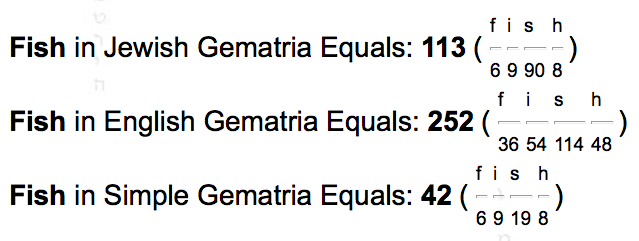## Monday, April 27, 2015

### 61 | Church, "Take Me to Church" and '61' Curiosities

I hate this song and it is played way too much.  Last night I made an interesting observation though.
• Church = 3+8+21+18+3+8 = 61
• Take = 2+1+2+5 = 10
• Me = 4+5 = 9
• to = 2+6 = 8
• Church = 3+8+3+9+3+8 = 34
• Take Me to Church = 61
Notice that Church sums to '61' in Simple English Gematria and 'Take Me to Church' sums to '61' in Pythagorean Gematria.
1. In the KJV Bible, the 61st Book has 61 verses.
2. The 18th 'Prime' Number is '61'.
• Prime = 16+18+9+13+5 = 61
3. Roger Maris set the MLB record with 61 HRs in '61
4. There were 61 Countries that participated in World War II
5. Sea of Galilee = 61 (The story of the miraculous catch from the Bible)
• The Sea of Galilee is where the 'Miraculous Catch' of 153 Fish takes place